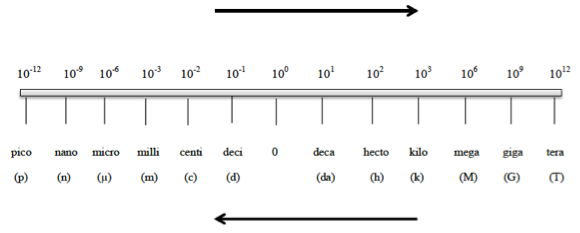# Problem: How many milligrams of sodium sulfate are equivalent to 0.0562 kg?a. 5.62 x 10-2 mgb. 5.62 x 104 mgc. 56.2 mgd. 5.62 x 1010 mge. 5.62 x 107 mg

80% (64 ratings)
###### FREE Expert Solution

We’re being asked to determine how many milligrams of sodium sulfate are equivalent to 0.0562 kg.

Metric Prefixes are “labels” that can be placed in front of base units. Whenever using the metric prefix chart, remember that “1” is associated with the metric prefix80% (64 ratings)###### Problem Details

How many milligrams of sodium sulfate are equivalent to 0.0562 kg?

a. 5.62 x 10-2 mg

b. 5.62 x 104 mg

c. 56.2 mg

d. 5.62 x 1010 mg

e. 5.62 x 107 mg

What scientific concept do you need to know in order to solve this problem?

Our tutors have indicated that to solve this problem you will need to apply the Metric Prefixes concept. You can view video lessons to learn Metric Prefixes. Or if you need more Metric Prefixes practice, you can also practice Metric Prefixes practice problems.

What is the difficulty of this problem?

Our tutors rated the difficulty ofHow many milligrams of sodium sulfate are equivalent to 0.05...as low difficulty.

How long does this problem take to solve?

Our expert Chemistry tutor, Sabrina took 1 minute undefined to solve this problem. You can follow their steps in the video explanation above.

What professor is this problem relevant for?

Based on our data, we think this problem is relevant for Professor Cordeiro's class at SMC.